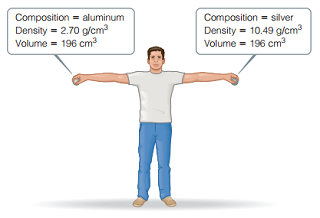# Problem: Consider the two spheres shown here, one made of silver and the other of aluminum. The spheres are dropped from a height of 2.1 m.What is the kinetic energy of the silver sphere at the moment it hits the ground? (Assume that energy is conserved during the fall and that 100% of the sphere’s initial potential energy is converted to kinetic energy by the time impact occurs.)

###### FREE Expert Solution

PE = 10.89 J

88% (457 ratings)###### Problem Details

Consider the two spheres shown here, one made of silver and the other of aluminum. The spheres are dropped from a height of 2.1 m.What is the kinetic energy of the silver sphere at the moment it hits the ground? (Assume that energy is conserved during the fall and that 100% of the sphere’s initial potential energy is converted to kinetic energy by the time impact occurs.)